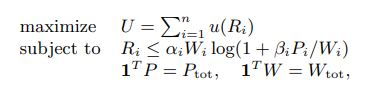# How to writre function "ylog(1+a/y)" where "y" is decision variable

Hi folks,

I am trying to solve the following problem with CVX:However, my decision variables are just “wi” and “Ri” and others are fixed known parameters (in the image “Pi” is also a decision variable). I have proven that it is convex.
I used two functions “rel_entr” and “inv_pos” to model the first constraint. I got the error as follows:

Disciplined convex programming error:
Cannot perform the operation: {real affine} .* {convex}

I would appreciate it if you can help me figure out the problem.
Thank you

The first constraint can be formulated as
`R <= -a*rel_entr(W,W+b*P)`

Note: I used b in place of beta, because `beta` is a MATLAB function.

Thank you very much. It helped me a lot.